``library(dplyr)``
``````##
## Attaching package: 'dplyr'``````
``````## The following objects are masked from 'package:stats':
##
##     filter, lag``````
``````## The following objects are masked from 'package:base':
##
##     intersect, setdiff, setequal, union``````
``````library(stringr)
library(ggplot2)
library(magrittr)``````
``````course_data = read.csv("UIUC Datasets/gpa/uiuc-gpa-dataset.csv")

Prepare data for analysis by calculating Average GPA and Student Enrollment for classes using the grade distribution as well as making some other minor adjustments

``````names(course_data)[7:20] = c("A+", "A", "A-", "B+", "B", "B-", "C+", "C", "C-", "D+", "D", "D-", "F", "W")

course_data\$Course_Number = paste(course_data\$Subject, course_data\$Number)

#Finds total num of students per class by computing sum of grade distributions
course_data\$students_enrolled = apply(course_data[, 7:20], MARGIN = 1, sum)

#Calculates average GPA per class using U of I's GPA weighting system
course_data\$Average_GPA = (4 * (course_data\$`A+`) + 4 * (course_data\$A) + 3.67 * (course_data\$`A-`) + 3.33 * (course_data\$`B+`) + 3 * (course_data\$B) + 2.67 * (course_data\$`B-`) + 2.33 * (course_data\$`C+`) + 2 * (course_data\$C) + 1.67 * (course_data\$`C-`) + 1.33 * (course_data\$`D+`) + 1 * (course_data\$D) + .67 * (course_data\$`D-`)) / (course_data\$students_enrolled - course_data\$W)

course_data\$Average_GPA = round(course_data\$Average_GPA, 2)
course_data\$Course.Title = as.character(course_data\$Course.Title)``````

Function that accepts a list of courses and returns a dataframe containing the average GPA for those courses

``````computeAvgGpa = function(courses) {
average_gpas = vector()

for (i in 1 : length(courses)) {
avg_grade = course_data %>% subset(Course.Title == courses[i]) %>% select(Average_GPA) %>% colMeans()
}

average_gpas = round(average_gpas, 2)
course_gpas = data.frame(courses, average_gpas)
course_gpas = arrange(course_gpas, desc(average_gpas))

return(course_gpas)
}``````

10 classes with the largest student enrollments over the past 7 years

``````unique_courses = unique(as.character(course_data\$Course.Title))

enrollments = vector(mode = "numeric")

for (i in 1 : length(unique_courses)) {
num_students = course_data %>% subset(Course.Title == unique_courses[i]) %>% select(students_enrolled) %>% sum()
enrollments = c(enrollments, num_students)
}

course_enrollments = data.frame(unique_courses, enrollments)
course_enrollments = arrange(course_enrollments, desc(enrollments))

ggplot(head(course_enrollments, 10), aes(x = unique_courses, y = enrollments, fill = enrollments)) +
geom_bar(stat = "identity") +
theme(axis.text.x = element_text(angle = 90, hjust = 1))``````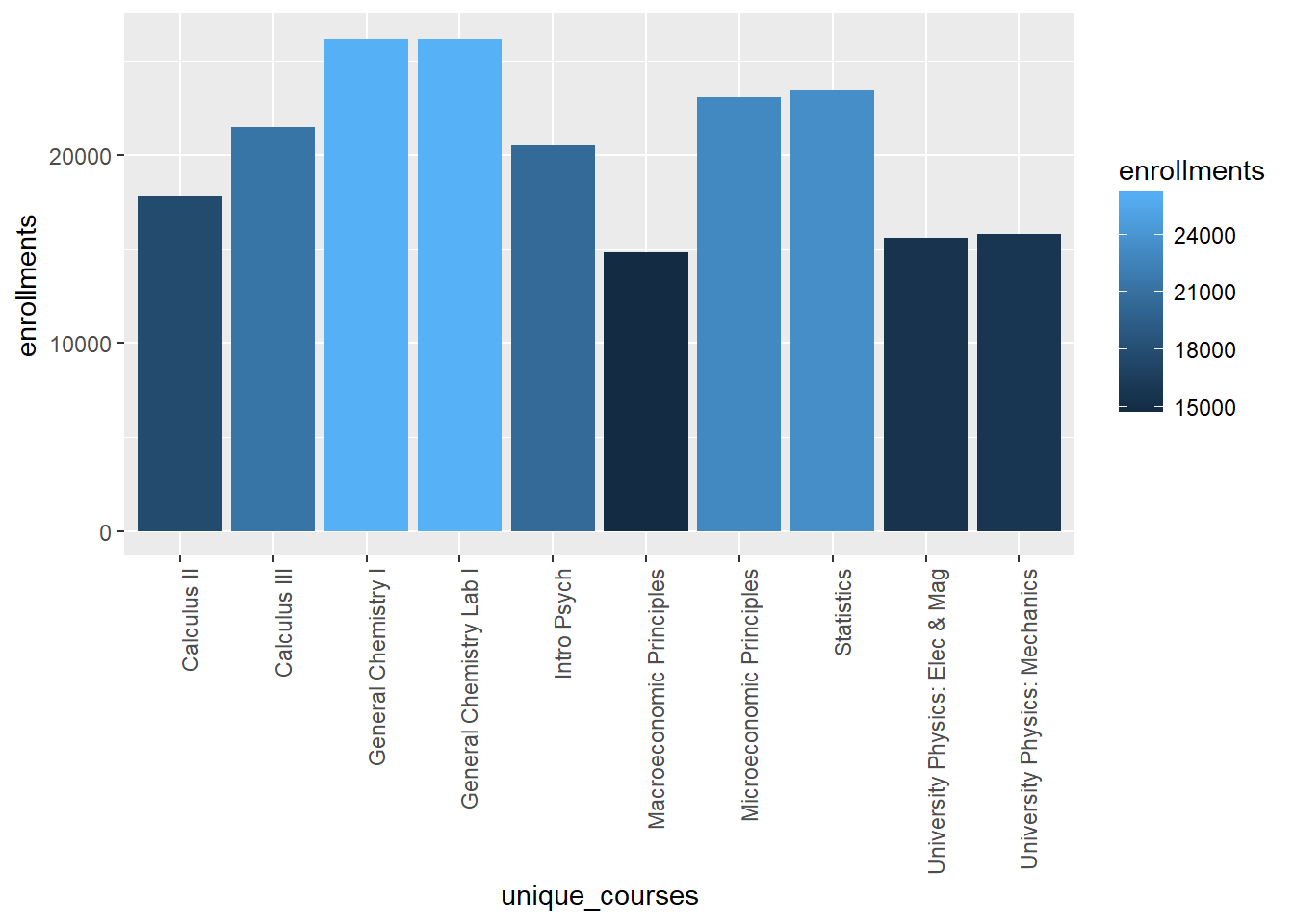10 Classes with the lowest average GPA over the past 7 years

``````courses_gpas = computeAvgGpa(unique_courses)

ggplot(data = tail(courses_gpas, 10), aes(x = courses, y = average_gpas)) +
geom_bar(stat = "identity", fill = "red") +
theme(axis.text.x = element_text(angle = 90, hjust = 1)) +
scale_y_continuous(breaks = seq(1, 4, by = .5), limits = c(0,4))``````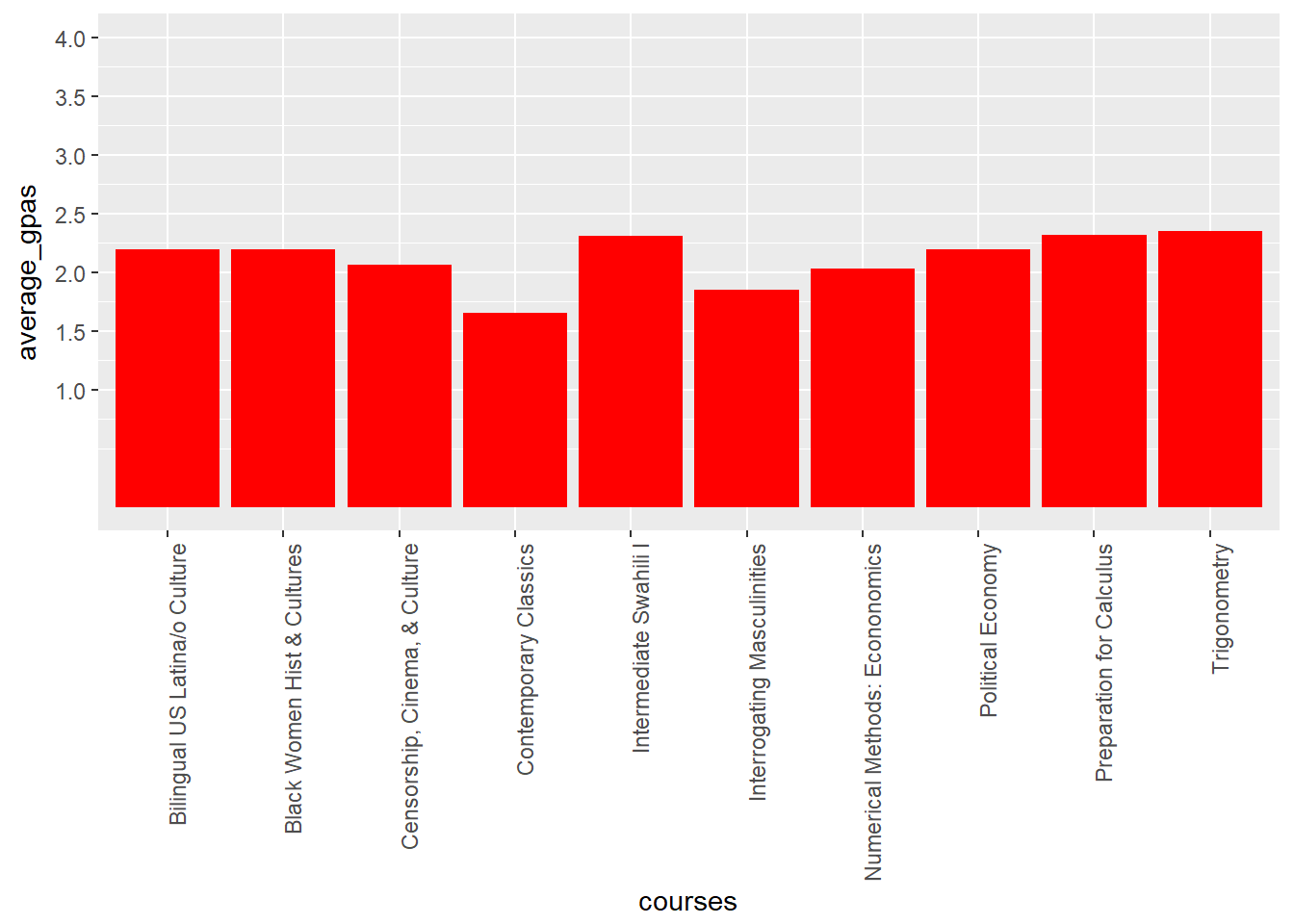The following classes all have average GPAs greater than 3.95

``courses_gpas %>% subset(average_gpas > 3.95) %>% select(courses)``
``````##                           courses
## 1                 Marching Illini
## 2                      Foal Watch
## 5  Prep for Recruitment Counselor
## 6              DGS Honors Seminar
## 7                  Illini Strings
## 8   Flavor Chemistry and Analysis
## 9  School Intrnshp Spch-Lang Path
## 10             Integrated Project
## 11         Harding Symphonic Band
## 12 Technology-Mgmt Honors Seminar
## 13     Undergrad Crop Sci Seminar
## 14 Cardiovascul/Critical Care Med
## 15  Food Safety and Public Health
## 16      Working With Farm Animals
## 17           Coaching for Success
## 18        Technical Communication
## 19         Cases Wealth Managment
## 20              Data Analytics II
## 21      Financial Statement Fraud
## 22   Global Capstone Project Trip
## 23      Technology & Mgmt Seminar
## 24         Companion Animal Rehab
## 25    Water in the Global Environ
## 26        Silicon Valley Workshop
## 27         Farm Food & Env Policy
## 28                   Black Chorus
## 29                    Capstone II
## 30  Spec Topics in Design Courses
## 31                         Jazz 1
## 32 Physical Activity Epidemiology
## 33  Computer-Assisted Instruction
## 34  Honors Symposium in Education
## 36                      Practicum
## 37      Strategic Operations Mgmt
## 38      Practical Problems in Atg
## 39   Researching Global Education
## 40  Freshman Materials Laboratory
## 42        Real Estate Development
## 43             Career Exploration
## 44      U of I Symphony Orchestra
## 45           Space System Seminar
## 46  Rethinking Ed: UGrad Research
## 47 Meteorological Instrumentation
## 48           China Immersion Trip
## 49     Optimiz in Computer Vision
## 50            Cultural Competence
## 51               Flavor Chemistry
## 53                   Storytelling
## 54    Swine Health and Production
## 55      Topics in LGBT Lit & Film
## 56   Small Fruits and Viticulture
## 57           Orchestral Repertory
## 58      Exploring Grapes and Wine
## 59                          Voice``````

10 majors that have the lowest average GPA for their classes and 10 majors with highest average GPA for their classes. (*NOTE - This analysis does not take a class’s enrollment numbers into account when calculating its impact on the major’s overall average class GPA. Thus, the results could be skewed by majors with extremely difficult classes that are only taken by a few students. Such a situation would cause this analysis to imply that the major is more difficult than it really is for the majority of its students who opt not to take those challenging courses)

``````unique_majors = unique(course_data\$Subject) %>% as.character()

for (i in 1 : length(unique_majors)) {
avg_grade = course_data %>% subset(Subject == unique_majors[i]) %>% select(Average_GPA) %>% colMeans()
}

geom_bar(stat = "identity", fill = "dark green") +
scale_y_continuous(breaks = seq(0, 4, by = .25))``````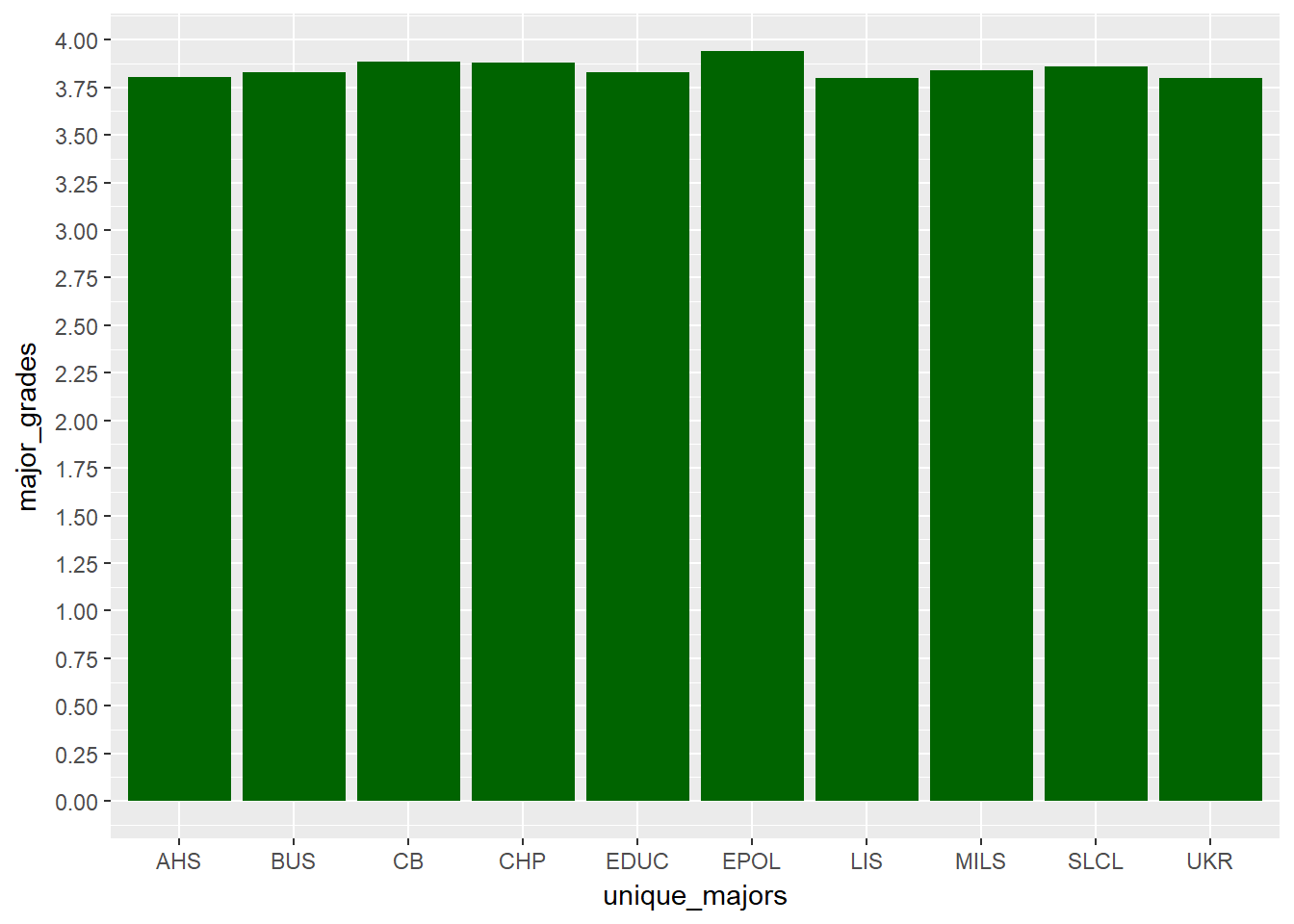``````ggplot(data = tail(major_gpas, 10), aes(x = unique_majors, y = major_grades)) +
geom_bar(stat = "identity", fill = "red") +
scale_y_continuous(breaks = seq(0, 4, by = .25), limits = c(0,4))``````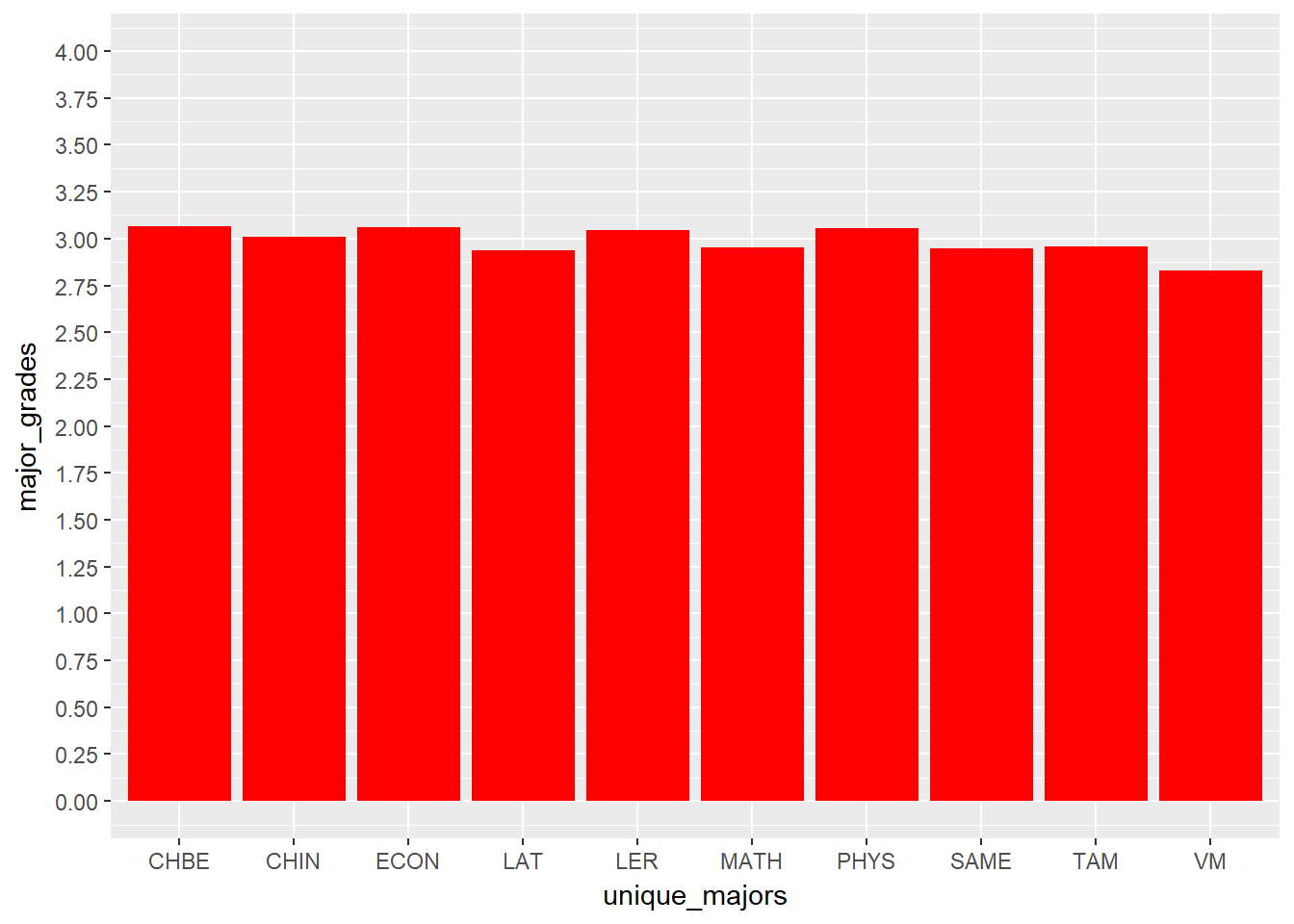10 majors that have the lowest average WEIGHTED GPA for their classes and the 10 majors that have the highest average WEIGHTED GPA for their classes. Weighted GPA takes into account a class’s enrollemnt numbers. Therefore, the impact of a class on a major’s weighted GPA is proportional to the number of students enrolled in that class.

``````weighted_grades = vector(mode = "numeric")

for (i in 1 : length(unique_majors)) {
subject_subset = course_data %>% subset(Subject == unique_majors[i])
avg_grade = sum(subject_subset\$Average_GPA * subject_subset\$students_enrolled) / sum(subject_subset\$students_enrolled)
}

geom_bar(stat = "identity", fill = "dark green") +
scale_y_continuous(breaks = seq(1,4, by = .25))``````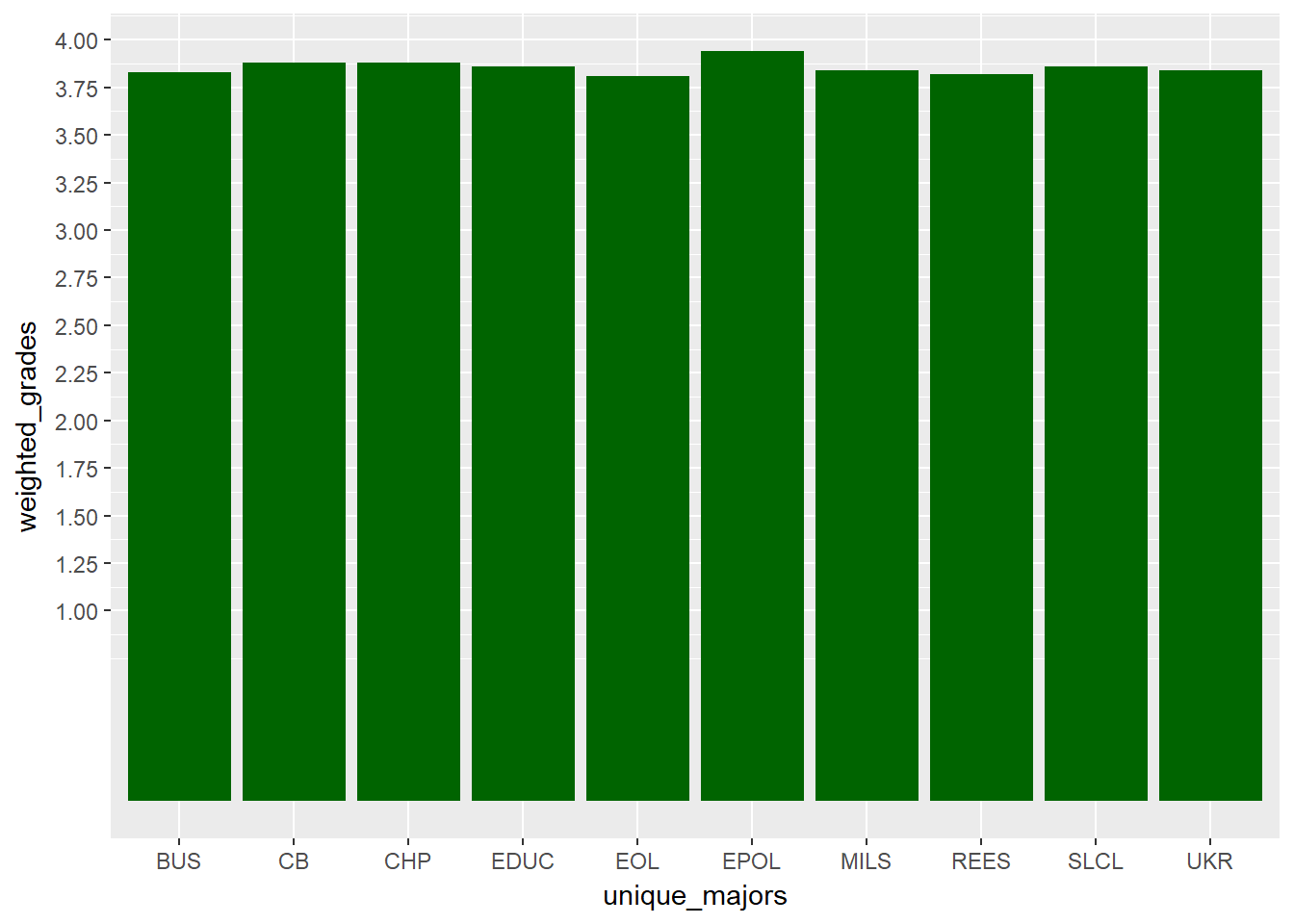``````ggplot(tail(weighted_major_gpas, 10), aes(x = unique_majors, y = weighted_grades)) +
geom_bar(stat = "identity", fill = "red") +
scale_y_continuous(breaks = seq(1,4, by = .25), limits = c(0,4))``````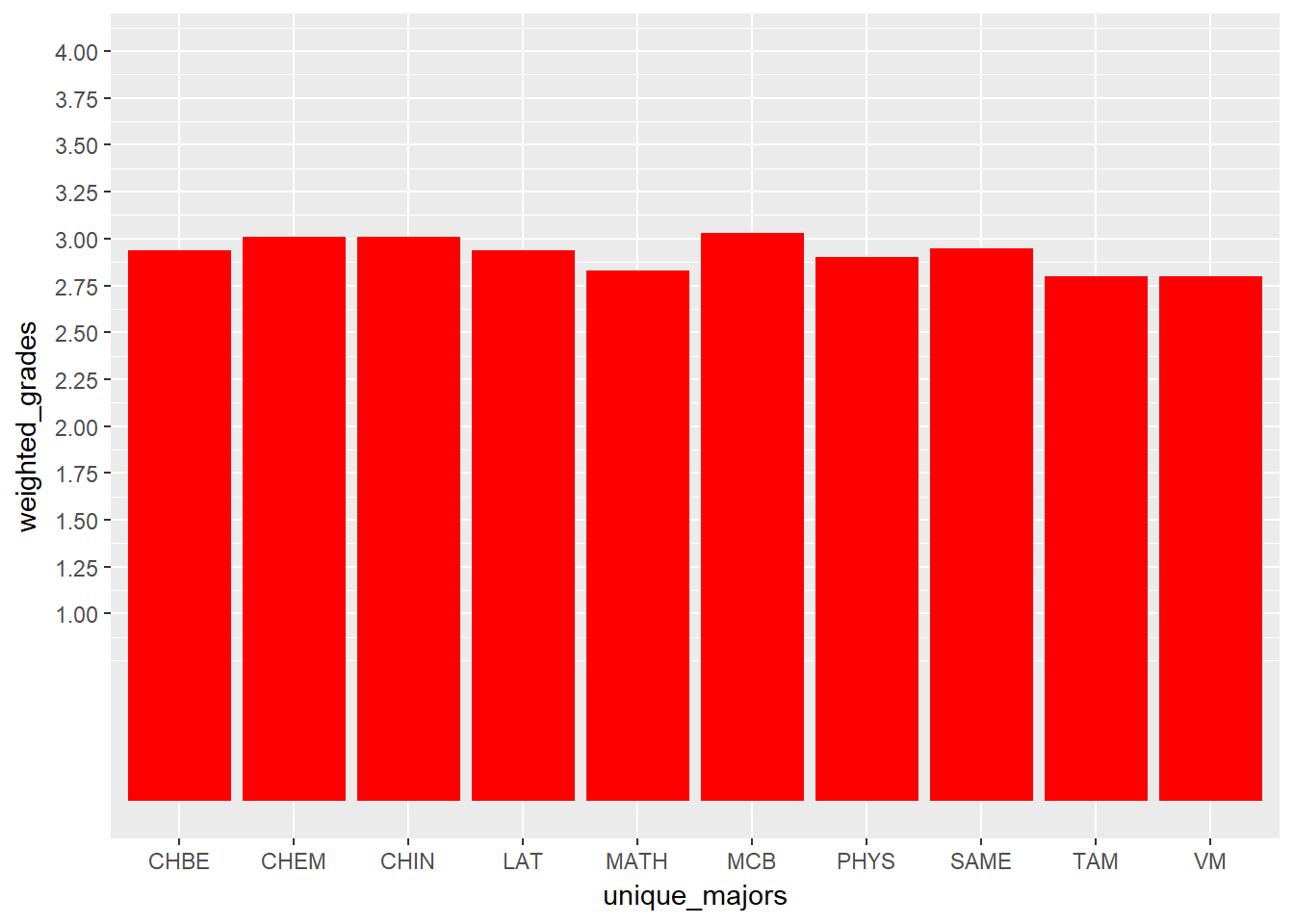Gen Ed classes with highest average GPA across different gen ed categories

``````adv_comp = gen_eds %>% subset(ACP == "ACP") %>% select(Course.Title) %>% unique()

non_western_minority = gen_eds %>% subset(CS == "NW" || CS == "US") %>% select(Course.Title) %>% unique()

western = gen_eds %>% subset(CS == "WCC") %>% select(Course.Title) %>% unique()

humanity_arts = gen_eds %>% subset(HUM == "HP" | HUM == "LA") %>% select(Course.Title) %>% unique()

natural_sciences = gen_eds %>% subset(NAT == "LS" | NAT == "PS") %>% select(Course.Title) %>% unique()

quant_reasoning1 = gen_eds %>% subset(QR == "QR1") %>% select(Course.Title) %>% unique()

quant_reasoning2 = gen_eds %>% subset(QR == "QR2") %>% select(Course.Title) %>% unique()

social_behavioral = gen_eds %>% subset(SBS == "BSC" || SBS == "SS") %>% select(Course.Title) %>% unique()

non_western_minority_gpas = computeAvgGpa(non_western_minority\$Course.Title)
western_gpas = computeAvgGpa(western\$Course.Title)
humanity_arts_gpas = computeAvgGpa(humanity_arts\$Course.Title)
natural_sciences_gpas = computeAvgGpa(natural_sciences\$Course.Title)
quant_reasoning1_gpas = computeAvgGpa(quant_reasoning1\$Course.Title)
quant_reasoning2_gpas = computeAvgGpa(quant_reasoning2\$Course.Title)
social_behavioral_gpas = computeAvgGpa(social_behavioral\$Course.Title)

``````##                           courses average_gpas
## 1    Senior Engineering Project I         3.89
## 2   Senior Engineering Project II         3.89
## 3                   Senior Thesis         3.85
## 4       Intro to Physics Research         3.77
## 5    Foundations of Education-ACP         3.76
## 6           Senior Design Project         3.73
## 7       Grimms' Fairy Tales - ACP         3.72
## 8  Foundations of Health Behavior         3.66
## 9          Ethics and Engineering         3.65
## 10           Principles Tech Comm         3.64``````
``print("\n")``
``##  "\n"``
``````ggplot(head(adv_comp_gpas, 10), aes(x = courses, y = average_gpas)) +
geom_bar(stat = "identity", fill = "blue") +
scale_y_continuous(breaks = seq(1,4, by = .25)) +
theme(axis.text.x = element_text(angle = 90, hjust = 1))``````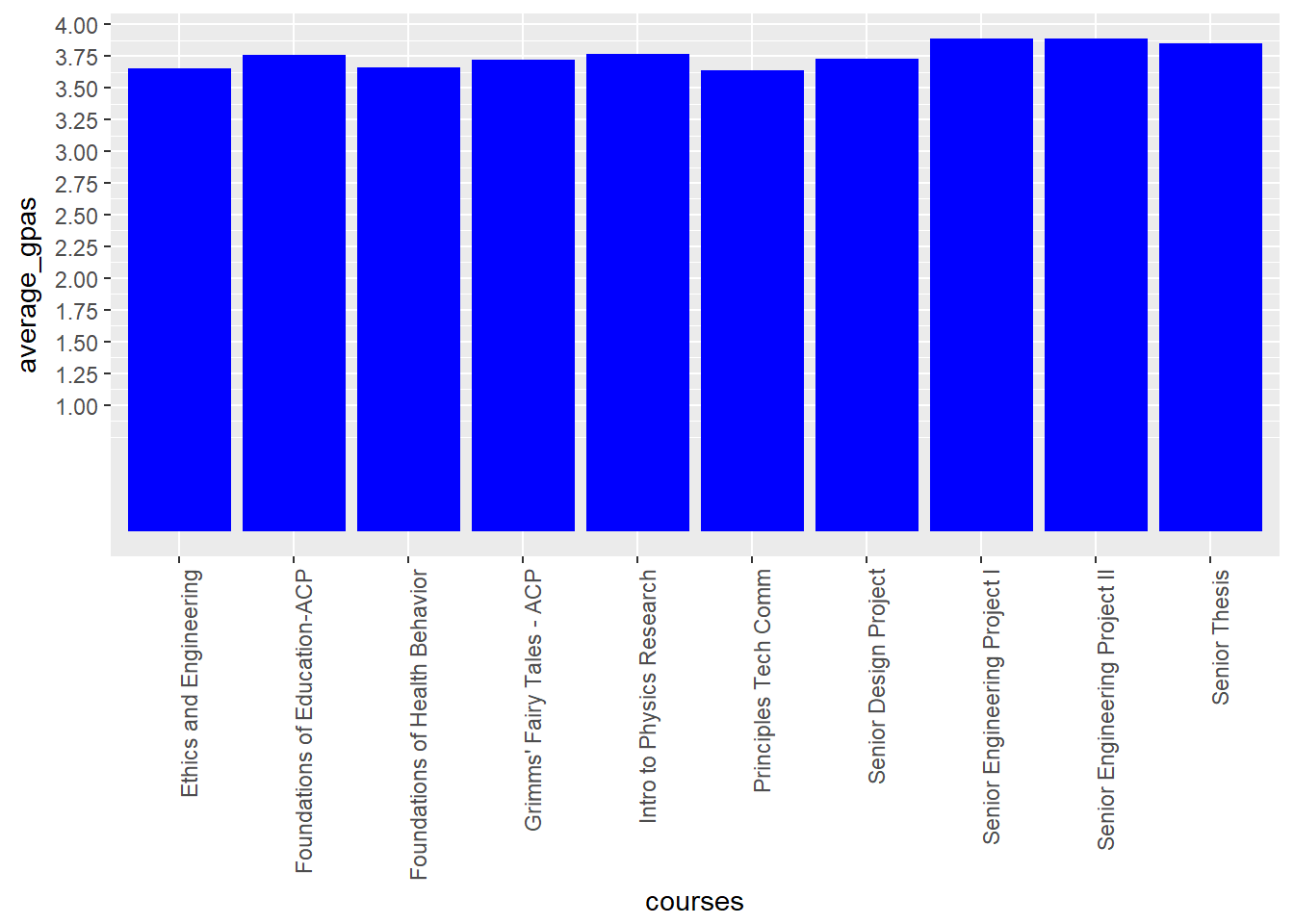``head(non_western_minority_gpas, 10)``
``````##                           courses average_gpas
## 1        Sculpture for Non-Majors         3.94
## 2    Intro to American Indian Lit         3.91
## 3                History of Korea         3.91
## 4              Sustainable Design         3.89
## 5    Senior Engineering Project I         3.89
## 6   Senior Engineering Project II         3.89
## 7        Cross-Cultural Thematics         3.88
## 8               Physics Made Easy         3.88
## 9  Intro Sustainable Food Systems         3.87
## 10        Introduction to Fashion         3.86``````
``print("\n")``
``##  "\n"``
``````ggplot(head(non_western_minority_gpas, 10), aes(x = courses, y = average_gpas)) +
geom_bar(stat = "identity", fill = "purple") +
scale_y_continuous(breaks = seq(1,4, by = .25)) +
theme(axis.text.x = element_text(angle = 90, hjust = 1))``````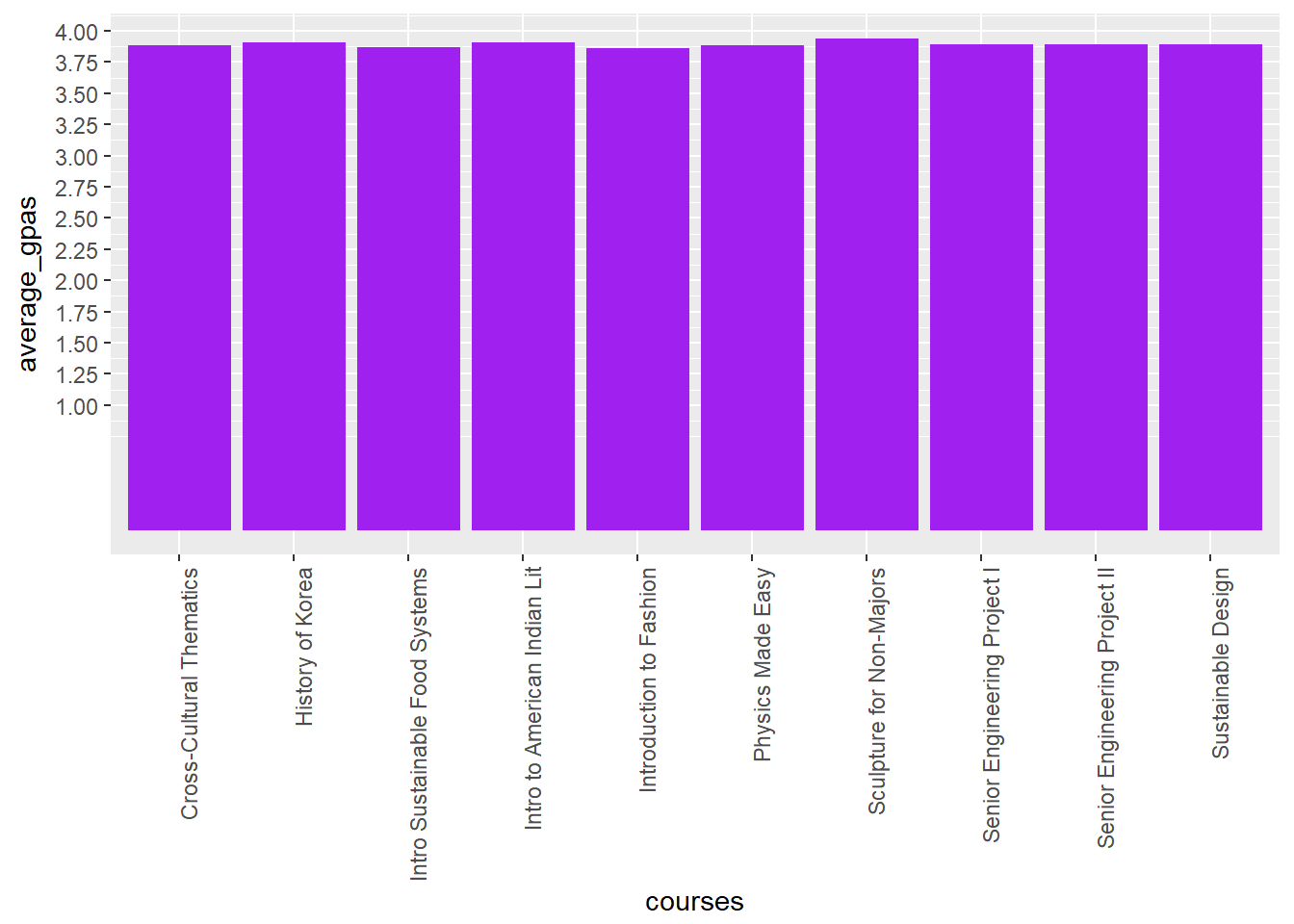``head(western_gpas, 10)``
``````##                           courses average_gpas
## 1               Ukrainian Culture         3.80
## 2     Intro to Contemporary Dance         3.75
## 3  Language, Technology & Society         3.75
## 4    Religion & Society in West I         3.73
## 5                  The Automobile         3.73
## 6       Grimms' Fairy Tales - ACP         3.72
## 8       Survey of American Lit II         3.69
## 9         Intro to Polish Culture         3.69
## 10  Religion & Society in West II         3.68``````
``print("\n")``
``##  "\n"``
``````ggplot(head(western_gpas, 10), aes(x = courses, y = average_gpas)) +
geom_bar(stat = "identity", fill = "turquoise") +
scale_y_continuous(breaks = seq(1,4, by = .25)) +
theme(axis.text.x = element_text(angle = 90, hjust = 1))``````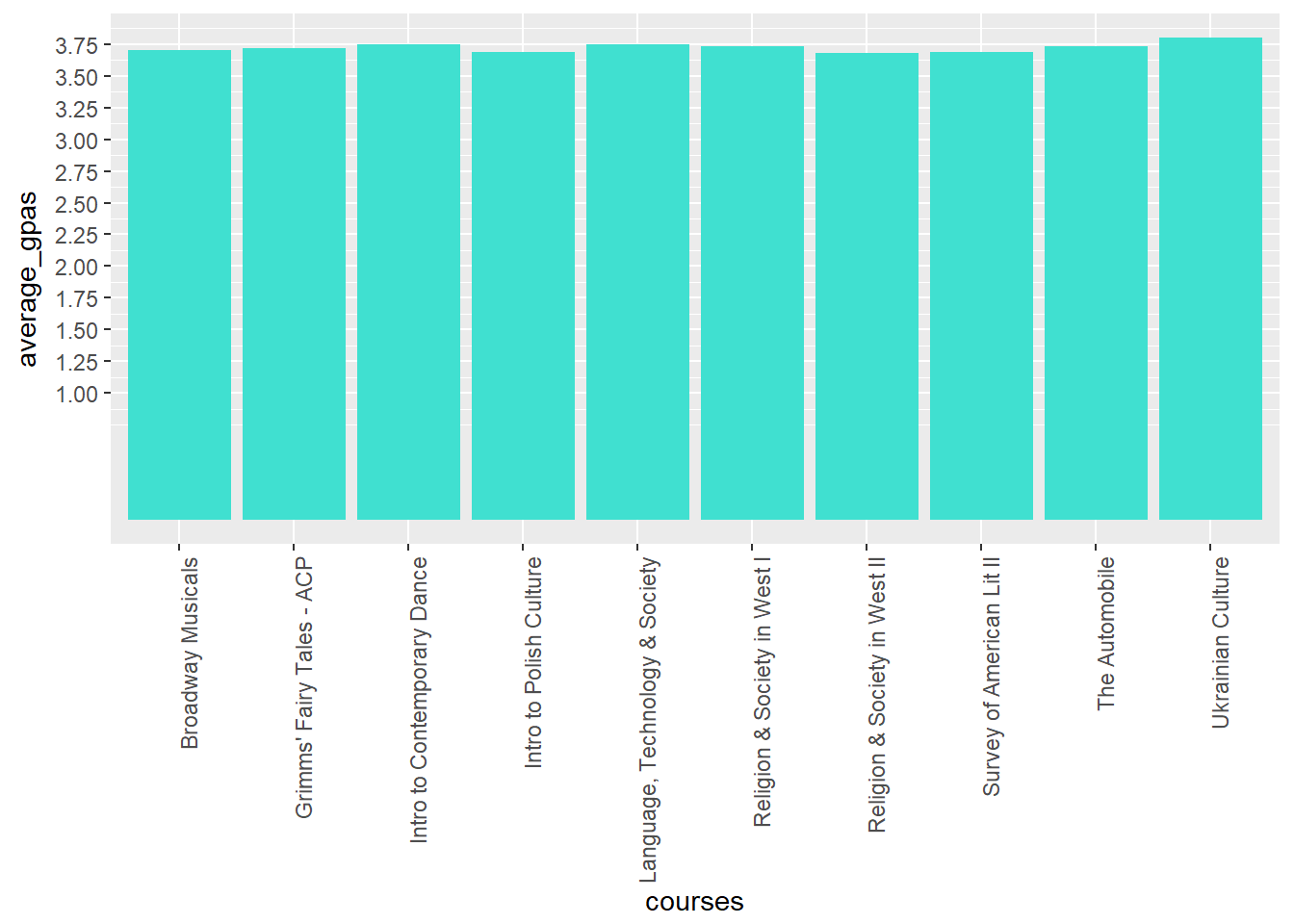``head(humanity_arts_gpas, 10)``
``````##                         courses average_gpas
## 1      Sculpture for Non-Majors         3.94
## 2  Intro to American Indian Lit         3.91
## 3              History of Korea         3.91
## 4            Sustainable Design         3.89
## 5      Cross-Cultural Thematics         3.88
## 6       Introduction to Fashion         3.86
## 7      Indian Cinema in Context         3.85
## 8       Painting for Non-Majors         3.82
## 9                 Text to Stage         3.80
## 10            Ukrainian Culture         3.80``````
``print("\n")``
``##  "\n"``
``````ggplot(head(humanity_arts_gpas, 10), aes(x = courses, y = average_gpas)) +
geom_bar(stat = "identity", fill = "navy blue") +
scale_y_continuous(breaks = seq(1,4, by = .25)) +
theme(axis.text.x = element_text(angle = 90, hjust = 1))``````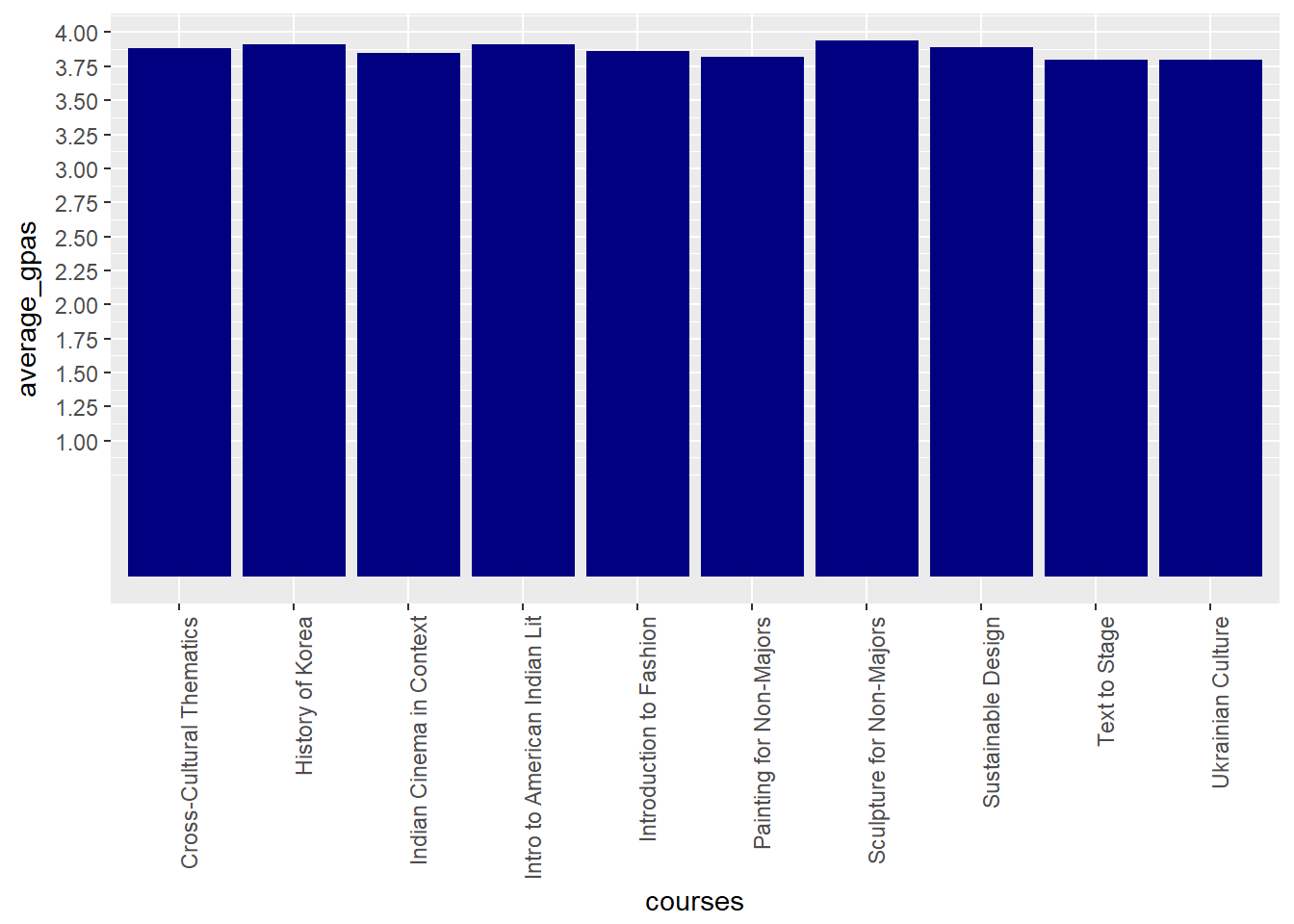``head(natural_sciences_gpas, 10)``
``````##                           courses average_gpas
## 1               Physics Made Easy         3.88
## 2  Intro Sustainable Food Systems         3.87
## 3    Severe and Hazardous Weather         3.78
## 4   Life With Animals and Biotech         3.76
## 5   Chemistry, Everyday Phenomena         3.71
## 6           Society and the Brain         3.65
## 7         General Chemistry Lab I         3.58
## 8        General Chemistry Lab II         3.58
## 9               Sustainable Earth         3.56
## 10      Biology of Human Behavior         3.51``````
``print("\n")``
``##  "\n"``
``````ggplot(head(natural_sciences_gpas, 10), aes(x = courses, y = average_gpas)) +
geom_bar(stat = "identity", fill = "violet") +
scale_y_continuous(breaks = seq(1,4, by = .25)) +
theme(axis.text.x = element_text(angle = 90, hjust = 1))``````